• +91 9971497814
• info@interviewmaterial.com

# Chapter 5- Laws of motion Interview Questions Answers

### Related Subjects

Question 1 :
Give the magnitude and direction of the net force acting on
(a) a drop of rain falling down with a constant speed,
(b) a cork of mass 10 g floating on water,
(c) a kite skillfully held stationary in the sky,
(d) a car moving with a constant velocity of 30 km/h on a rough road,
(e) a high-speed electron in space far from all material objects, and free of electric and magnetic fields.

(a) Zero net force
The rain drop is falling with a constant speed. Hence, it acceleration is zero. As per Newton’s second law of motion, the net force acting on the rain drop is zero.
(b) Zero net force
The weight of the cork is acting downward. It is balanced by the buoyant force exerted by the water in the upward direction. Hence, no net force is acting on the floating cork.
(c) Zero net force
The kite is stationary in the sky, i.e., it is not moving at all. Hence, as per Newton’s first law of motion, no net force is acting on the kite.
(d) Zero net force
The car is moving on a rough road with a constant velocity. Hence, its acceleration is zero. As per Newton’s second law of motion, no net force is acting on the car.
(e) Zero net force
The high speed electron is free from the influence of all fields. Hence, no net force is acting on the electron.

Question 2 :
A pebble of mass 0.05 kg is thrown vertically upwards. Give the direction and magnitude of the net force on the pebble,
(a) during its upward motion,
(b) during its downward motion,
(c) at the highest point where it is momentarily at rest. Do your answers change if the pebble was thrown at an angle of 45° with the horizontal direction?

0.5 N, in vertically downward direction, in all cases
Acceleration due to gravity, irrespective of the direction of motion of an object, always acts downward. The gravitational force is the only force that acts on the pebble in all three cases. Its magnitude is given by Newton’s second law of motion as:
F = m × a
Where,
F = Net force
m = Mass of the pebble = 0.05 kg
a = g = 10 m/s2
∴F = 0.05 × 10 = 0.5 N
The net force on the pebble in all three cases is 0.5 N and this force acts in the downward direction.
If the pebble is thrown at an angle of 45° with the horizontal, it will have both the horizontal and vertical components of velocity. At the highest point, only the vertical component of velocity becomes zero. However, the pebble will have the horizontal component of velocity throughout its motion. This component of velocity produces no effect on the net force acting on the pebble.

Question 3 :

Givethe magnitude and direction of the net force acting on a stone of mass 0.1 kg,

(a)just after it is dropped from the window of a stationary train,

(b)just after it is dropped from the window of a train running at a constantvelocity of 36 km/h,

(c) just after it is dropped from the windowof a train accelerating with 1 m s–2,

(d) lying on the floor of a train which isaccelerating with 1 m s–2, the stone being at rest relative to thetrain. Neglect air resistance throughout.

(a)1 N; vertically downward

Mass of the stone, m = 0.1kg

Acceleration of the stone, a =g = 10 m/s2

Asper Newton’s second law of motion, the net force acting on the stone,

F = ma = mg

=0.1 × 10 = 1 N

Accelerationdue to gravity always acts in the downward direction.

(b)1 N; vertically downward

Thetrain is moving with a constant velocity. Hence, its acceleration is zero inthe direction of its motion, i.e., in the horizontal direction. Hence, no forceis acting on the stone in the horizontal direction.

Thenet force acting on the stone is because of acceleration due to gravity and italways acts vertically downward. The magnitude of this force is 1 N.

(c)1 N; vertically downward

It is given that the train is acceleratingat the rate of 1 m/s2.

Therefore, the net force acting on thestone, F‘ = ma = 0.1 × 1 = 0.1 N

This force is acting in the horizontaldirection. Now, when the stone is dropped, the horizontal force F,’stops acting on the stone. This is because of the fact that the force acting ona body at an instant depends on the situation at that instant and not onearlier situations.

Therefore,the net force acting on the stone is given only by acceleration due to gravity.

F = mg = 1 N

Thisforce acts vertically downward.

(d)0.1 N; in the direction of motion of thetrain

Theweight of the stone is balanced by the normal reaction of the floor. The onlyacceleration is provided by the horizontal motion of the train.

Acceleration of the train, a =0.1 m/s2

Thenet force acting on the stone will be in the direction of motion of the train.Its magnitude is given by:

F = ma

=0.1 × 1 = 0.1 N

Question 4 :
One end of a string of length l is connected to a particle of mass m and the other to a small peg on a smooth horizontal table. If the particle moves in a circle with speed v the net force on the particle (directed towards the centre) is:
(i) T, (ii)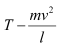, (iii)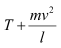, (iv) 0
T is the tension in the string. [Choose the correct alternative].

When a particle connected to a stringrevolves in a circular path around a centre, the centripetal force is providedby the tension produced in the string. Hence, in the given case, the net forceon the particle is the tension T, i.e.,

T =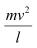Where F isthe net force acting on the particle.

Question 5 :

A constant retarding force of 50 N isapplied to a body of mass 20 kg moving initially with a speed of 15 ms–1.How long does the body take to stop?

Retarding force, F = –50 N

Mass of the body, m = 20 kg

Initial velocity of the body, u =15 m/s

Final velocity of the body, v =0

Using Newton’s second law of motion, theacceleration (a) produced in the body can be calculated as:

F = ma

–50 = 20 × a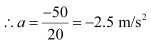Using the first equation of motion, the time(t) taken by the body to come to rest can be calculated as:

v = u + at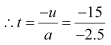=6 s

Question 6 :

A constant force acting on a body of mass3.0 kg changes its speed from 2.0 m s–1 to 3.5 m s–1 in25 s. The direction of the motion of the body remains unchanged. What is themagnitude and direction of the force?

0.18N; in the direction of motion of the body

Mass of the body, m = 3 kg

Initial speed of the body, u =2 m/s

Final speed of the body, v =3.5 m/s

Time, t = 25 s

Using the first equation of motion, theacceleration (a) produced in the body can be calculated as:

v = u + at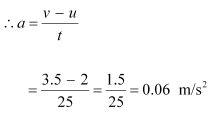Asper Newton’s second law of motion, force is given as:

F = ma

=3 × 0.06 = 0.18 N

Sincethe application of force does not change the direction of the body, the netforce acting on the body is in the direction of its motion.

Question 7 :

Abody of mass 5 kg is acted upon by two perpendicular forces 8 N and 6 N. Givethe magnitude and direction of the acceleration of the body.

2 m/s2, at an angle of 37° with aforce of 8 N

Mass of the body, m = 5 kg

Thegiven situation can be represented as follows: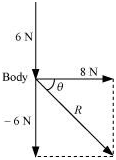Theresultant of two forces is given as: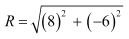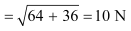θ is the angle made by R withthe force of 8 N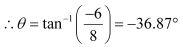The negative sign indicates that θ isin the clockwise direction with respect to the force of magnitude 8 N.

As per Newton’s second law of motion, theacceleration (a) of the body is given as:

ma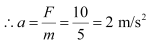Question 8 :

Thedriver of a three-wheeler moving with a speed of 36 km/h sees a child standingin the middle of the road and brings his vehicle to rest in 4.0 s just in timeto save the child. What is the average retarding force on the vehicle? The massof the three-wheeler is 400 kg and the mass of the driver is 65 kg.

Initial speed of the three-wheeler, u =36 km/h = 10 m/s

Final speed of the three-wheeler, v =0 m/s

Time, t = 4 s

Mass of the three-wheeler, m =400 kg

Mass of the driver, m‘ = 65 kg

Total mass of the system, M =400 + 65 = 465 kg

Using the first law of motion, theacceleration (a) of the three-wheeler can be calculated as:

v = u + at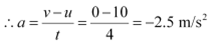Thenegative sign indicates that the velocity of the three-wheeler is decreasingwith time.

UsingNewton’s second law of motion, the net force acting on the three-wheeler can becalculated as:

F = Ma

=465 × (–2.5) = –1162.5 N

Thenegative sign indicates that the force is acting against the direction ofmotion of the three-wheeler.

Question 9 :

A rocket with a lift-off mass 20,000 kg isblasted upwards with an initial acceleration of 5.0 m s–2. Calculatethe initial thrust (force) of the blast.

Mass of the rocket, m =20,000 kg

Initial acceleration, a = 5m/s2

Acceleration due to gravity, g =10 m/s2

UsingNewton’s second law of motion, the net force (thrust) acting on the rocket isgiven by the relation:

F – mg = ma

F = m (g + a)

=20000 × (10 + 5)

=20000 × 15 = 3 × 105 N

Question 10 :

A body of mass 0.40 kg moving initially witha constant speed of 10 m s–1 to the north is subject to aconstant force of 8.0 N directed towards the south for 30 s. Take the instantthe force is applied to be = 0, the position of the body atthat time to be = 0, and predict its position at t =–5 s, 25 s, 100 s.

Mass of the body, m = 0.40kg

Initial speed of the body, u =10 m/s due north

Force acting on the body, F =–8.0 N

Accelerationproduced in the body,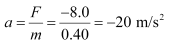(i) At t = –5 s

Acceleration, a‘ = 0 and u =10 m/s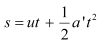=10 × (–5) = –50 m

(ii) At t = 25 s

Acceleration, a” = –20 m/s2 and u =10 m/s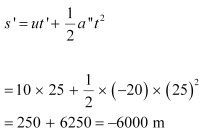(iii) At t = 100 s

For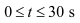a = –20 m/s2

u = 10 m/s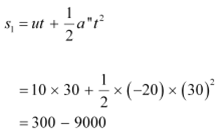=–8700 m

For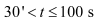As per the first equation of motion,for t = 30 s, final velocity is given as:

v = u + at

=10 + (–20) × 30 = –590 m/s

Velocityof the body after 30 s = –590 m/s

Formotion between 30 s to 100 s, i.e., in 70 s: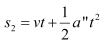=–590 × 70 = –41300 m

Total distance,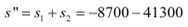=–50000 m

Todays Deals### Chapter 5- Laws of motion Contributorskrishan

Name:
Email:

# Latest News# 9000 interview questions in different categories